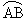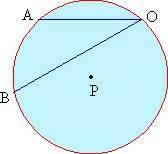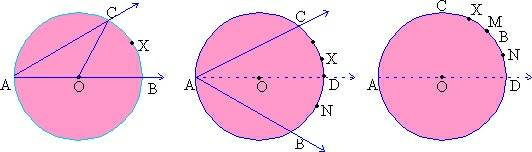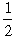Home MonkeyNotes Printable Notes Digital Library Study Guides Message Boards Study Smart Parents Tips College Planning Test Prep Fun Zone Help / FAQ How to Cite New Title Request

 7.4 Inscribed angles Whereas central angles are formed by radii, inscribed angles are formed by chords. As shown in figure 8.5 the vertex o of the inscribed angle AOB is on the circle. The minor arccut on the circle by an inscribed angle is called as the intercepted arc.Figure 7.5 Theorem: The measure of an inscribed angle is half the measure of its intercepted arc. Proof: For a circle with center O Ð BAC is the inscribed angle and arc BXC is the intercepted arc. To prove that m Ð BAC = 1/2 m (arc BXC). There arise three cases as shown in figure 8.6 (a), 8.6 (b) and 8.6 (c).Click here to enlarge     Figure 7.6 (a)        Figure 7.6 (b)       Figure 7.6 (c) Case 1: The center is on the angle figure 7.6 (a) join c to O. D OAC is an isosceles triangle as seg.OA = seg.OC. Assume m Ð OAC = m Ð OCA = P m Ð COA = 180 - 2 P as sum of all the angles of a triangle is 1800. Ð COA & Ð COB form a linear pair. Therefore they are supplementary. m Ð COB = 1800 - m Ð COA = 1800 - ( 1800 - 2P) = 2P = 2 m Ð BAC But m Ð BAC = m (arc BXC) \ m Ð BAC =m (arc BXC) Case 2: Figure 7.6 (b). The center is in the interior of the angle. in this case let D be the other end point of the diameter drawn through A. Let arc CMD be intercepted by Ð CAD and let arc Ð BND be that which is intercepted by Ð DAB. From case 1 m Ð CAD =m ( arc CMD) and m Ð DAB =m (arc BND) m Ð CAD + m Ð DAB =m (arc CMD) +m (arc BND) m Ð BAC ={ m ( arc CMD) + m (arc BND) } =m (arc BXC) Case 3: The center is in the exterior of the angle. Again let D be the other end point of the diameter drawn through A. Let arc CMD be the one intercepted by Ð CAD and let arc BND be the one intercepted by Ð DAB. From case 1 m Ð CAD =m (arc CMD) m Ð DAB =m (arc BND) m Ð CAD - m Ð DAB =m (arc CMD) -m (arc BND) m Ð CAB ={ M (arc CMD) - m (arc BND) } = m (arc BXC) Index 7.1 Introduction 7.2 Lines of circle 7.3 Arcs 7.4 Inscribed angels 7.5 Some properties od tangents, secants and chords 7.6 Chords and their arcs 7.7 Segments of chords secants and tangents 7.8 Lengths of arcs and area of sectors Chapter 8
 Search: All Products Books Popular Music Classical Music Video DVD Toys & Games Electronics Software Tools & Hardware Outdoor Living Kitchen & Housewares Camera & Photo Cell Phones Keywords: